Courses

Test: Precipitation - 2

10 Questions MCQ Test GATE Civil Engineering (CE) 2022 Mock Test Series | Test: Precipitation - 2

Description
Attempt Test: Precipitation - 2 | 10 questions in 30 minutes | Mock test for Civil Engineering (CE) preparation | Free important questions MCQ to study GATE Civil Engineering (CE) 2022 Mock Test Series for Civil Engineering (CE) Exam | Download free PDF with solutions
QUESTION: 1

The Double mass curve technique is adopted to

Solution:

If the conditions relevant to the recording of a raingauge station have undergone a significant change during the period of record, inconsistency would arise in the rainfall data of that station. The checking for inconsistency of record is done by the double mass curve technique. This technique is based on the principal that when each recorded data comes from the same parent population, they are consistent.

QUESTION: 2

Solution:
QUESTION: 3

The mass curve of rainfall of a storm is a plot of

Solution:
QUESTION: 4

The probable maximum depth of precipitation over a catchment is given by the relation PMP =

Solution:

The Probable Maximum Precipitation (PMP) is defined as the greatest or extreme rainfall for a given duration that is physically possible over a station or basin. Statistical studies indicate that PMP can be estimated as,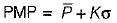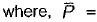mean of annual maximum rainfall series, σ = standard deviation of the series and K = a frequency factor which depends upon the statistical distribution of the series, number of years of record and the return period.

QUESTION: 5

A plot between rainfall intensity versus time is called as

Solution:
QUESTION: 6

By DAD analysis the maximum average depth over an area of 104 km2 due to one-day storm is found to be 47 cm. For the same area the maximum average depth for a three day storm can be expected to be

Solution:

The maximum depth for a given storm decreases with the area. For a given area the maximum depth increases with the duration.

QUESTION: 7

A hyetograph is a piot of

Solution:
QUESTION: 8

For a given storm the highest rainfall P0 and the average rainfall dept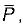are related as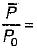Solution:

For a rainfall of a given duration, the average depth decreases with the area in an exponential fashion given by,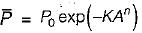where,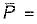average depth in cms over an area A km2
P0 = highest amount of rainfall in cm at the storm
K and n are constants for a given region.

QUESTION: 9

An isohyet is a line joining points having

Solution:
QUESTION: 10

The Thiessen polygon is

Solution:Use Code STAYHOME200 and get INR 200 additional OFF Use Coupon Code

Track your progress, build streaks, highlight & save important lessons and more!

Similar ContentRelated tests